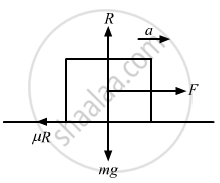Advertisement Remove all ads

# A Car Starts from Rest on a Half Kilometre Long Bridge. the Coefficient of Friction Between the Tyre and the Road is 1.0. Show that One Cannot Drive Through the Bridge in Less than 10 S. - Physics

Sum

A car starts from rest on a half kilometre long bridge. The coefficient of friction between the tyre and the road is 1.0. Show that one cannot drive through the bridge in less than 10 s.

Advertisement Remove all ads

#### Solution

Let a be the maximum acceleration of the car for crossing the bridge.From the above diagram,
ma = μR
(For more accelerations the tyres will slip)
ma = μmg
a = μg = 1 × 10 = 10 m/s2
To cross the bridge in minimum possible time, the car must be at its maximum acceleration.
u = 0, s = 500 m, a = 10 m/s2
From the equation of motion,
s=ut+1/2at^2
Substituting respective values
500=0+(1/2)10t^2

=>t=sqrt100=10s

Therefore, if the car's acceleration is less than 10 m/s2, it will take more than 10 s to cross the bridge. So, one cannot drive through the bridge in less than 10 s.

Is there an error in this question or solution?
Advertisement Remove all ads

#### APPEARS IN

HC Verma Class 11, 12 Concepts of Physics 1
Chapter 6 Friction
Exercise | Q 17 | Page 98
Advertisement Remove all ads
Advertisement Remove all ads
Share
Notifications

View all notifications

Forgot password?